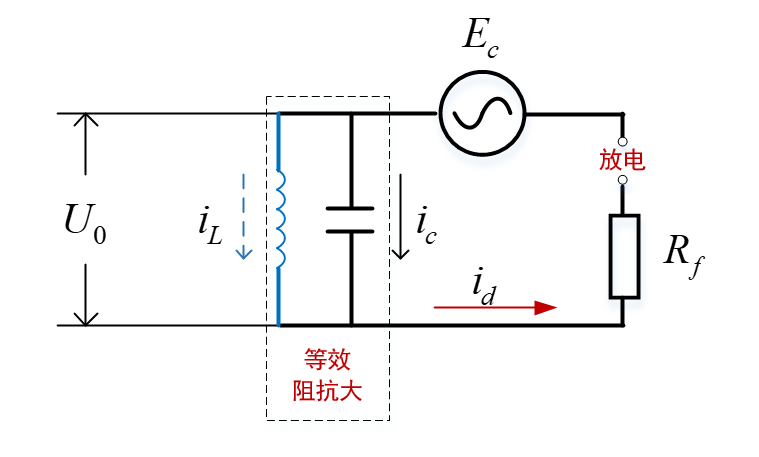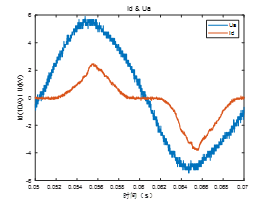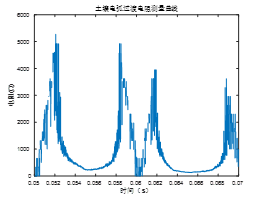# 1. 土壤放电的本质是辉光放电1. 此放电持续时间长，一个完整的放电周期是工频的半个周波，即包括零休时间是10ms，如果去掉零休时间也达到6~7ms。
2. 在放电的过程中，电压和电流不断的发生变化，即引起辉光放电的场强经历从小变大又从大变小的过程。
3. 接地介质为土壤，土壤是一种导体，但导电性并没有导体那么强，这导致了其有一定的散流电阻，在散流过程中的发热导致其发生热电离，即先导放电，接地点电压越高，先导放电效应越强烈，会向土壤内部深入的更深。
4. 又由于土壤本身的导电性，包裹等离子体的未充分电离的土壤能够导电，这使得电场分布与空气放电不同，没有绝对意义上的地，这种先导放电即不能同空气放电一样发展下去，也不会发生主放电。
5. 先导放电所形成的等离子体通道，相当于向土壤内部插入一根金属导体，这使得从接地点位置看进去的接地电阻变小了，先导放电形成的等离子体通道在电压最大时刻附近最深，等效电阻最小，而此电阻由于串联在电力系统的零序回路中，会反过来影响接地点的电压，其本质上是一种场路的耦合系统。

# 2. 土壤先导放电发展模型

## 2.1 能量输入

$W_c = \beta W_t \tag{1}$

β 是先导位移所需能量占全部能量的系数。 0 < β ≤ 1 。

$W _ { c } = \frac { 1 } { 2 } \rho \pi r _ { j } ^ { 2 } d l _ { j } v _ { j } ^ { 2 } \tag{2}$

$\sum _ { j = 1 } ^ { n } \frac { 1 } { 2 } ( \rho \pi r _ { j } ^ { 2 } d l _ { j } ) v _ { j } ^ { 2 } = \beta \sum _ { j = 1 } ^ { n } q _ { j } E _ { j } d l _ { j } \tag{3}$

$v _ { j } ^ { 2 } = \frac { 2 \beta } { \pi r _ { j } ^ { 2 } \rho } ( \int i _ { j } d t ) E _ { j } \tag{4}$

## 2.2 先导发展的平均速度

$t _ { j } = t _ { p j } + t _ { 0 j } \tag{5}$

$v _ { a l } = \frac { 1 } { \sum _ { j = 1 } ^ { n } t _ { j } } \sum _ { j = 1 } ^ { n } ( v _ { p j } t _ { p j } + v _ { 0 j } t _ { 0 j } ) \tag{6}$

$v _ { p j } ^ { 2 } = \frac { 2 } { \pi r _ { j } ^ { 2 } } \frac { \beta } { \rho } q _ { p j } E _ { j } \tag{7}$ $v _ { 0 j } ^ { 2 } = \frac { 2 } { \pi r _ { j } ^ { 2 } } \frac { \beta } { \rho } q _ { 0 j } E _ { j } \tag{8}$

$v _ { a l } = \frac { 1 } { \sum _ { j = 1 } ^ { n } t _ { j } } \sum _ { j = 1 } ^ { n } [ ( \frac { 2 } { \pi r _ { j } ^ { 2 } } \frac { \beta } { \rho } E _ { j } ) ^ { 1 / 2 } ( q _ { p j } ^ { 1 / 2 } t _ { p j } + q _ { 0 j } ^ { 1 / 2 } t _ { 0 j } ) ] \tag{9}$

$v_{0j}$通常相对于$v_{pj}$可以忽略不计。 $v _ { 0 j }$是在时间$t_{0}$期间先导发展的平均速度。

$v _ { a l } = ( \frac { 2 } { \pi r _ { j } ^ { 2 } } \frac { \beta } { \rho } E _ { j } ) ^ { 1 / 2 } ( \frac { 1 } { \sum _ { j = 1 } ^ { n } t _ { j } } \sum _ { j = 1 } ^ { n } ( q _ { p j } ^ { 1 / 2 } t _ { p j } + q _ { 0 j } ^ { 1 / 2 } t _ { 0 j } ) ) \tag{10}$

$v _ { a l } \cong ( \frac { 2 } { \pi r _ { j } ^ { 2 } } \frac { \beta } { \rho } E _ { j } ) ^ { 1 / 2 } ( \frac { 1 } { \sum _ { j = 1 } ^ { n } t _ { j } } \sum _ { j = 1 } ^ { n } ( q _ { p j } ^ { 1 / 2 } t _ { p j } ) ) \tag{11}$

$E_j$和/或 $q_{pj}$ 越高，先导发展的平均速度就越高。

## 2.3 等离子体的密度

$p V = \frac { m } { M } R T \tag{12}$

$\rho = \frac { M } { R } \frac { p } { T } \tag{13}$

$\rho ( kg m ^ { - 3 } ) = \frac { k } { T ( K ) R } \tag{14}$

## 2.4 先导发展头部电场计算

$E _ { j } = \frac { 2 U _ { j } } { R _ { e } \operatorname { ln } [ 4 ( \frac { D - L _ { z } } { R _ { e } } ) ] } \tag{15}$

$L_z$和 D 分别是前导轴向长度和间隙长度，$U_j = U − \Delta U_j$是先导尖端上的电压（其中 U 是电极上施加的电压，$\Delta U_j$是沿通道的电压降）和 $R_e$ 等效电极的半径。

$R_e$ 可以表示为等离子长度的线性关系

$R _ { e } = \alpha L_z \tag{16}$

## 2.5 先导放电的平均速度计算

$v _ { a l } = ( \frac { 4 \beta T _ { 0 } U _ { j } R } { \pi r _ { 0 } ^ { 2 } k R _ { e } \operatorname { ln } [ 4 ( \frac { D - L _ { z } } { R _ { e } } ) ] } ) ^ { 1 / 2 } \times ( \frac { 1 } { \sum _ { j = 1 } ^ { n } t _ { j } } \sum _ { j = 1 } ^ { n } ( q _ { p j } ^ { 1 / 2 } t _ { p j } ) ) \tag{19}$